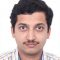Home » Core Java » Math.round Java ExampleHe is a Software Engineer working in an industry-leading organization. He has completed his bachelors from Visweswaraya Technological University. In his career, he has worked in designing and implementing various software systems involving Java/J2EE, Spring/ Spring Boot, React JS, JQuery, Hibernate and related database technologies. He loves to share his knowledge and always look forward to learning and explore new technologies. He loves to spend his free time with his family. He enjoys traveling and loves to play cricket.

# Math.round Java Example

The Math.round Java – java.lang.math – library contains the static methods to perform basic numeric mathematical operations. Math.round is one such method, which returns the nearest whole number to the argument. In this article, we will dig deeper into this method.

## 1. What does rounding mean?

Rounding is the mathematical process of making the number up or down to the nearest whole number. In this number process, any given decimal number is converted to the nearest whole number. For example,

1. 3.2 is rounded to 3
2. 3.7 is rounded to 4
3. 3.5 is rounded to 4
4. 7.49 is rounded to 7
5. 7.4999999 is rounded to 7

Note: When the first digit after the decimal point is less than 5, then the number is rounded to lower integer and when it is 5 or more, then it is rounded to the next integer. Another important thing to remember is, not to double round. Do not round 7.49 as 7.5 and then round 7.5 to 8. Remember that, 7.49 is always rounded to 7.

## 2. Math.round Java examples

Math.round is a static method and is part of java.lang.Math class. This method performs the rounding of a floating-point number to the nearest integer or long. There are two overloaded implementations of this method,

1. `static int round(float a)` returns the closest integer to the argument.
2. `static long round(double a)` returns the closest long to the argument.

Both of these methods always round up.

### 2.1. Code examples

Below example shows how math round works. It covers both regular scenarios and edge cases.

```public class MathRoundingDemo {
public static void main(String[] args) {
//Round the floating point number to integer
//Case 1: Rounding the number to lower whole number
System.out.println("Rounding the number to lower whole number");
System.out.println(String.format("3.2 is rounded to - %d", Math.round(3.2)));
System.out.println("---------------------------------------------------");

//Case 2: Rounding the number number to next integer
System.out.println("Rounding the number number to next integer");
System.out.println(String.format("3.7 is rounded to - %d", Math.round(3.7)));
System.out.println("---------------------------------------------------");

//Case 3: When the only number after decimal point is 5, number is rounded to upper whole number
System.out.println("When the only number after decimal point is 5, number is rounded to upper whole number");
System.out.println(String.format("3.5 is rounded to - %d", Math.round(3.5)));
System.out.println("---------------------------------------------------");

//Case 4: Numbers never double round up
System.out.println("Numbers never double round up");
System.out.println(String.format("7.4999 is rounded to - %d", Math.round(7.4999)));
System.out.println("---------------------------------------------------");

//Rounding to long value
System.out.println("Rounding to long value");
long roundedToLong = Math.round(123234.5);
System.out.println("Rounded long value - " + roundedToLong);
System.out.println("---------------------------------------------------");

//Rounding the edge case numbers
//Case 1: When argument passed is not a number, then ZERO is returned
System.out.println("When argument passed is not a number, then ZERO is returned");
System.out.println(String.format("0/0 is rounded to - %d", Math.round(Float.NaN)));
System.out.println("---------------------------------------------------");

//Case 2: When negetive infinity is rounded then Long.MIN_VALUE is returned
float negativeInfinity = -1/0.0f;
int roundedNum = Math.round(negativeInfinity);
System.out.println("When negetive infinity is rounded then Long.MIN_VALUE is returned");
System.out.println(String.format("-1/0 is rounded to - %d", roundedNum));
System.out.println("---------------------------------------------------");

//Case 2: When positive infinity is rounded then Long.MAX_VALUE is returned
float positiveInfinity = 1/0.0f;
int roundedMaxNum = Math.round(positiveInfinity);
System.out.println("When positive infinity is rounded then Long.MAX_VALUE is returned");
System.out.println(String.format("1/0 is rounded to - %d", roundedMaxNum));
System.out.println("---------------------------------------------------");
}
}
```

The output of the program looks as below:

### 2.2. How to run the sample program

• Save example code to `MathRoundingDemo.java` in a directory of your choice.
• Open the command prompt and navigate to the directory where the file is saved.
• Run the command `javac MathRoundingDemo.java` to compile, this will generate `MathRoundingDemo.class`.
• To run the example, run command `java MathRoundingDemo`. Don’t specify any extension.

### 2.3. Edge cases

• When argument passed is a NaN, then it returns ZERO
• When the passed number argument is negative infinity, it returns Long.MIN_VALUE
• When the argument is positive infinity, it returns Long.MAX_VALUE

## 3. Why do we need to use rounding

• Rounding numbers makes it simpler and easier to use. Precision isn’t needed always. Precision makes the calculations complicated.
• Comes handy whenever an approximation is required
• It is applicable when we have to provide counts

However, there is a caveat. Rounding is less accurate. Wherever precise results are expected (Ex: Banking calculations, Scientific calculations) do not use rounding.

You can download the full source code of this example here: Math.round Java Example(+4 rating, 4 votes)
Start the discussion Views Tweet it!

# Do you want to know how to develop your skillset to become a Java Rockstar?

## Subscribe to our newsletter to start Rocking right now!

### and many more ....